• A
• A
• A
• АБB
• АБB
• АБB
• А
• А
• А
• А
• А
Обычная версия сайта
Меню
Найдено 14 публикаций
Сортировка:
по названию
по году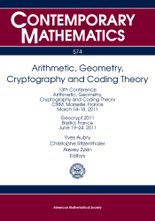Книга
Iss. 574. Providence: American Mathematical Society, 2012.

Том содержит труды 13й конференции AGCT и конференции Geocrypt. Темы конференций - различные аспекты арифметической и алгебраической геометрии, теории чисел, теории кодирования, криптографии. Основные направления, обсуждавшиеся на конференциях, включают в себя теорию кривых над конечными полями, теорию абелевых многообразий над глобальными и конечными полями, теорию дзета и L-функций, асимптотические проблемы в теории чисел и алгебраической геометрии, алгоритмические вопросы теории кривых и абелевых многообразий, теорию кодов, исправляющих ошибки, в особенности, теорию алгебро-геометрических кодов, криптографические вопросы, связанные с кривыми и абелевыми многообразиями.

Добавлено: 3 января 2013Книга
Bogachev V. Providence: American Mathematical Society, 2010.

The book gives a systematic account of the theory of differentiable measures and the Malliavin calculus.

Добавлено: 5 марта 2014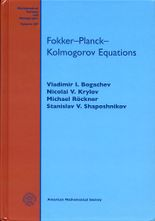Книга
Bogachev V., Krylov N. V., Roeckner M. et al. Providence: American Mathematical Society, 2015.

This book gives an exposition of the principal concepts and results related to second order elliptic and parabolic equations for measures, the main examples of which are  Fokker-Planck-Kolmogorov equations for stationary and transition probabilities of diffusion  processes. Existence and uniqueness of solutions are studied along with existence  and Sobolev regularity of their densities and upper and lower bounds for the latter. The target readership includes mathematicians and physicists whose research is related to diffusion processes as well as elliptic and parabolic equations.

Добавлено: 25 февраля 2016Книга
Bogachev V. Providence: American Mathematical Society, 1998.

This book presents a systematic exposition of the modern theory of Gaussian measures. The basic properties of finite and infinite dimensional Gaussian distributions, including their linear and nonlinear transformations, are discussed. The book is intended for graduate students and researchers in probability theory, mathematical statistics, functional analysis, and mathematical physics. It contains a lot of examples and exercises. The bibliography contains 844 items; the detailed bibliographical comments and subject index are included.

Добавлено: 10 марта 2014Книга
Под редакцией: I. M. Krichever, V. M. Buchstaber. American Mathematical Society, 2008.

This volume contains a selection of papers based on presentations given in 2006-2007 at the S. P. Novikov Seminar at the Steklov Mathematical Institute in Moscow. Novikov's diverse interests are reflected in the topics presented in the book. The articles address topics in geometry, topology, and mathematical physics. The volume is suitable for graduate students and researchers interested in the corresponding areas of mathematics and physics.

Добавлено: 18 апреля 2014Книга
Providence: American Mathematical Society, 2005.

Idempotent mathematics is a rapidly developing new branch of the mathematical sciences that is closely related to mathematical physics. The existing literature on the subject is vast and includes numerous books and journal papers. A workshop was organized at the Erwin Schrodinger Institute for Mathematical Physics (Vienna) to give a snapshot of modern idempotent mathematics. This volume contains articles stemming from that event. Also included is an introductory paper by G. Litvinovand additional invited contributions. The resulting volume presents a comprehensive overview of the state of the art. It is suitable for graduate students and researchers interested in idempotent mathematics and tropical mathematics.

Добавлено: 29 апреля 2013Книга
V.A.Vassiliev. Providence: American Mathematical Society, 2001.
Добавлено: 10 февраля 2018Книга
Shen A., Uspensky V. A., Vereshchagin N. American Mathematical Society, 2017.
Добавлено: 12 октября 2017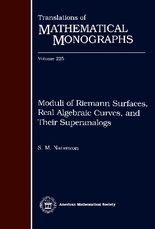Книга
Natanzon S. M. Vol. 225. American Mathematical Society, 2004.

The space of all Riemann surfaces (the so-called moduli space) plays an important role in algebraic geometry and its applications to quantum field theory. The present book is devoted to the study of topological properties of this space and of similar moduli spaces, such as the space of real algebraic curves, the space of mappings, and also superanalogs of all these spaces. The book can be used by researchers and graduate students working in algebraic geometry, topology, and mathematical physics.

Добавлено: 13 апреля 2021Книга
Polishchuk A., Positselski L. Providence: American Mathematical Society, 2005.
Добавлено: 13 октября 2011Книга
Vol. 621. American Mathematical Society, 2014.
Добавлено: 17 апреля 2017Книга
Под науч. редакцией: V. Buchstaber, B. Dubrovin, I. M. Krichever. Providence: American Mathematical Society, 2014.
Добавлено: 15 сентября 2016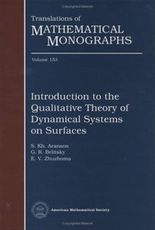Книга
Aranson S., Belitsky G., Zhuzhoma E. V. Vol. 153: Introduction to the qualitative theory of dynamical systems on surfaces. American Mathematical Society, 1996.

The book is an introduction to the qualitative theory of dynamical systems on manifolds of low dimension (on the circle and on surfaces). Along with classical results, it reflects the most significant achevements in this area obtained in recent times. The reader of this book need to be familiar only with basic courses in differential equations and smooth manifolds.

Добавлено: 2 октября 2014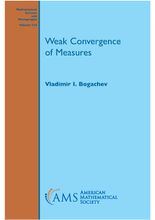Книга
Bogachev V. American Mathematical Society, 2018.
Добавлено: 15 ноября 2019